### Stationary perturbation theory, degenerate states

#### Problem:

Consider a two-dimensional infinite potential square well of width L,
(U = 0 for 0 < x, y < L, U = infinite everywhere else) with an added perturbation

H' = g sin(2πx/L)sin(2πy/L).

(a)  Calculate the first order perturbation to the ground state energy eigenvalue.
(b)  Calculate the first order perturbation to the first excited state energy eigenvalue.

Solution:

• Concepts:
Stationary perturbation theory for non-degenerate and for degenerate states
• Reasoning:
The ground state of two-dimensional infinite potential square well is not degenerate.  The first excited state is two-fold degenerate.
• Details of the calculation:
H = H0 + H'.
The eigenfunctions of H0 are Φnl(x,y) = (2/L)sin(nπx/L)sin(lπy/L), with eigenvalues
E = (n2 + l22ħ2/(2mL2).
(a)  For the ground state n = l = 1.  The  ground state is not degenerate.
The unperturbed ground state energy is E00 = π2ħ2/(mL2).  The first order perturbation correction is
E10 = <Φ11|H'|Φ11> = (g4/L2)∫0Lsin2(πx/L) sin2(πy/ L)sin(2πx/L) sin(2πy/L)dxdy.
= (4g/L2)∫0Lsin2(πx/L) sin(2πx/L)dx)∫0Lsin2(πy/L) sin(2πy/L)dy = 0.

(b)  The first excited state is two-fold degenerate.
We can have n = 2, l = 1, or n = 1, l = 2.
We have to diagonalize the matrix of H' in the subspace spanned by these two degenerate states.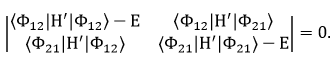12|H'|Φ12> = (4g/L2)∫0Lsin2(πx/L)sin(2πx/L)dx)∫0Lsin2(2πy/L) sin(2πy/L)dy = 0.
21|H'|Φ21> = (4g/L2)∫0Lsin2(2πx/L)sin(2πx/L)dx)∫0Lsin2(πy/L) sin(2πy/L)dy = 0.
12|H'|Φ21> = (4g/L2)∫0Lsin(πx/L) sin2(2πx/L)dx)∫0Lsin2(2πy/L) sin(πy/L)dy = C
C = (4g/π2)(∫ 0πsin(x) sin2(2x)dx)2 = (4g/π2)*64/225.
12|H'|Φ21> = (4g/L2)∫0Lsin(πx/L) sin2(2πx/L)dx)∫0Lsin2(2πy/L) sin(πy/L)dy = C
E2 - C2 = 0.  E11 = ±C.  The perturbation removes the degeneracy.

#### Problem:

A particle of mass m is trapped in a 3-dimensional, infinite square well.
V(x,y,z) = 0, if x, y, and z are less than a and not negative,
V(x,y,z) = ∞, otherwise.
(a)  What are the two lowest energy eigenvalues?  Are these energies degenerate?
(b)  The box is placed into a uniform gravitational field with gravitational acceleration g in the negative z-direction.  Use perturbation theory to calculate first-order corrections to the energy eigenvalues from part (a).

Solution:

• Concepts:
Three-dimensional square well potentials, perturbation theory
• Reasoning:
We are supposed to find first order energy corrections to the two lowest energy eigenvalues of the 3D square well.
• Details of the calculation:
(a)  The eigenfunctions and eigenvalues of the 3D infinite square well are
Φnml(x,y,z) = (2/a)3/2sin(kxx)sin(kyy)sin(kzz),  with kx = πn/a,  ky = πq/a,  kz = πl/a, n, q, l = 1, 2, ... .
The associated eigenvalues are
Enql = (n2 + q2 + l22ħ2/(2ma2) = (kx2 + ky2 + kz22/(2m).
(b)  The two lowest energy eigenvalues are
E01 = E111 = 3π2ħ2/(2ma2),  E02 = E112 = E121 = E211 = 6π2ħ2/(2ma2).
The eigenvalue  E02 is three-fold degenerate.
(b)  The perturbation is W = mgz.
E11 = <Φ111|W|Φ111> = (2/a)3mg∫0asin2(πx/a)dx ∫0asin2(πy/a)dy ∫0az sin2(πz/a)dz
= (2/a)3mg(a/2)4 = mga/2 = energy correction for the ground state.

To find the corrections to E02 and to find out if the degeneracy is removed by the perturbation, we have to diagonalize W in the subspace spanned by Φ211, Φ121, and Φ112.
211|W|Φ211> = (2/a)3mg∫0asin2(2πx/a)dx ∫0asin2(πy/a)dy ∫0az sin2(πz/a)dz = mga/2.
121|W|Φ121> = mga/2.
112|W|Φ112> = (2/a)3mg∫0asin2(πx/a)dx ∫0asin2(πy/a)dy ∫0az sin2(2πz/a)dz = mga/2.
All the off-diagonal matrix elements of W are zero.  The perturbation does not remove the degeneracy.
E12 = mga/2.

#### Problem:

Consider a charged particle on a ring of unit radius with flux Φ/Φ0 = a passing through the ring, where Φ0 = h/(2e) is the flux quantum.  The Hamiltonian operator can be written as H = H0 + U, where
H0 = (i∂/∂θ + α)2  and U = U0 cosθ.
θ is the angular coordinate.  We have chosen units with ħ = 2m = 1.
(a)  Find the complete set of eigenvalues and eigenfunctions of H0.
(b)  Use perturbation theory to find the first and second-order corrections to the ground state energy E0 of H0 due to the perturbation U for 0 < α < ½ .
(c)  For α = ½ the ground state energy of H0 is degenerate.  Find the first-order correction to E0 for this case.

Solution:

• Concepts:
First and second order perturbation theory for non-degenerate states, first order perturbation theory for degenerate states.
• Reasoning:
We find the eigenvalues of H0, which are non-degenerate if α ≠ ½.  Perturbation theory for nondegenerate states therefore gives the energy corrections.  For α = ½ the ground state is degenerate and perturbation theory for degenerate states must be used.
• Details of the calculation:
(a)  H0ψ(θ) = Eψ(θ).
Try ψ(θ) = Nexp(ibθ),  H0exp(ibθ) = (-b + α)2exp(ibθ).
ψ(θ) = ψ(θ + 2π) -->  exp(ibθ) = exp(ib(θ + 2π)) -->  b = n, n = 0, ±1, ±2, ... .
ψ(θ) = Nexp(inθ),  En = (-n + α)2,
0|ψ|2dθ = N20dθ  = 1 -->  N = (2π).

(b)  Let 0 < α < ½.  Then all states are non degenerate and the ground state has n = 0.
E0 = E00 + E10 + E20 + ...,  E00 = α2.  E10 = <ψ0|H'|ψ0> = (2π)U00cosθ dθ = 0.

E20 = ∑n≠0 |<ψn|H'|ψ0>|2/(E00 - E0n).
0|H'|ψ0> = (2π)-1U00cosθ exp(inθ)dθ
= (4π)-1U00exp(i(n+1)θ)dθ + (4π)-1U00exp(i(n-1)θ)dθ
= δn,-1 U0/2 +  δn,+1 U0/2.
E20 = (U02/4)/(E00 - E0-1) + (U02/4)/(E00 - E01)
= -(U02/4)[1/(1 + 2α) + 1/(1 - 2)α] = -U02/(2 - 8α2)

(c)  If α = ½ then all states are degenerate.  The ground state is any linear combination of n = 0 and n = 1.
We have to diagonalize the matrix of U in the subspace spanned by
ψ0(θ) = (2π) and ψ1(θ) = (2π) exp(iθ).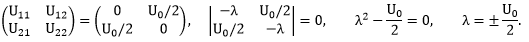E10 = ±U0/2.
E10 = U0/2, Φ = (1/√2)(ψ0(θ) + ψ1(θ)),  E10 = -U0/2, Φ = (1/√2)(ψ0(θ) - ψ1(θ)).

#### Problem:

Calculate the splitting induced among the degenerate n = 2 levels of a hydrogenic atom, when this atom is placed in a uniform electric field E pointing in the z-direction.  This is the linear Stark effect.  You may use the following explicit hydrogenic wave functions |nlm>.

|200> = (4π)(2a)-3/2(2 - r/a)exp(-r/2a),
|211> = (8π)(2a)-3/2(r/a) exp(-r/2a) sinθ e,
|210> = (4π)(2a)-3/2(r/a) exp(-r/2a) cosθ,
|21-1> = (8π)(2a)-3/2(r/a) exp(-r/2a) sinθ e-iφ.

0e-br rn dr = n!/bn+1.
Hint: Exploit symmetries!

Solution:

• Concepts:
First order perturbation theory, degenerate states
• Reasoning:
The uniform electric field changes the energy of the proton and the electron and therefore perturbs the Hamiltonian.
• Details of the calculation:
H = H0 + qe|E|z, where z = (ze - zp).
The energy of the proton in the field is -∫0zpqe|E|dz = -qe|E|zp.
The energy of the electron in the field is ∫0zeqe|E|dz = qe|E|ze.
The potential energy of both particles is -qe|E|(zp - ze) = qe|E|z = -p∙E.
Let us use first-order perturbation theory.
The first excited state is 4-fold degenerate.
E02 = μe4/(8ħ2).
To find E12 we have to diagonalize the operator qe|E|z = qe|E|r cosθ in the subspace spanned by |200>, |210>, |211>, and |21-1>.  The matrix elements between states with the same parity are zero.  This implies that all diagonal matrix elements are zero and all elements between states with the same l are zero.  The matrix elements between states with different m are zero.  The only matrix elements left are <210|z|200> and <200|z|210>.
<210|z|200> = qe|E|(4π)-1(2a)-30dφ∫0πcos2θ sinθ dθ ∫0r3dr (r/a)(2 - r/a) exp(-r/a)
= -3qe|E|a.
We therefore have to diagonalize the matrix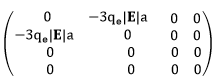in the |200>, |210>, |211>, |21-1> basis.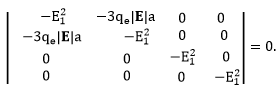(E12)2[(E12)2  - (3qe|E|a)2] = 0.

We have (E12)2 = 0 (two fold degenerate ), or  (E12)2 = (3qe|E|a)2, E12 = ±(3qe|E|a).
The eigenvectors corresponding to E12 = 0 are |211> and |21-1>.
The eigenvector corresponding to E12 = 3qe|E|a is  (1/√2)(|210> - |200>).
The eigenvector corresponding to E12 = -3qe|E|a is (1/√2)(|210> + |200>).
The four fold degeneracy is changed into two non degenerate eigenvalues and one two fold degenerate eigenvalue.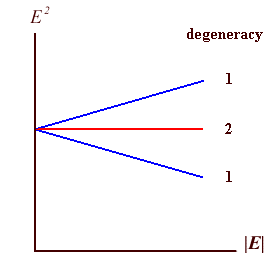#### Problem:

Positronium is a bound state of an electron and a positron.
(a)  What is the energy eigenvalue for the 1s state?
(b)  If the spin-spin interaction is neglected, what is the degree of degeneracy of the ground state?
(c)  Assume that the spin-spin interaction is given by ASeSp, where Se and Sp are the spin operators for the electron and positron respectively, and A is a coupling constant.  Show that the ground state is split into two states and find the degree of degeneracy of those states.

Solution:

• Concepts:
Hydrogenic atoms, a system of two spin ½ particles.
• Reasoning:
Positronium is a hydrogenic atom.  It is a bound state of two spin ½ particles.  The wave function in coordinate space and the energy levels can be obtained from the wave function and energy levels of the hydrogenic atom using scaling rules.
• Details of the calculation:
(a)  For the hydrogen atom we have En = -EI/n2
For a hydrogenic atom we have En = -EI'/n2, where EI' = Z2EI(μ'/μ).
In this problem μ' = me-me+/(me- + me+) = me/2 , Z = 1.
Therefore E0 = -13.6/2 eV.
E0 is the energy of the 1s (ground) state.
(b)  The spins of the two particles can combine to form the S = 1 (triplet) and S = 0 (singlet) state.  The ground state is therefore 4-fold degenerate.
(c)  The energy shift due to the spin-spin interaction is given by
A <S,Ms|SeSp|S,Ms>.  SeSp= ½(S2 - Se2 - Sp2).
SeSp|S,Ms> = (ħ2/2)(S(S+1) - ½(3/2) - ½(3/2))|S,Ms> = (ħ2/2)(S(S+1) - (3/2))|S,Ms>.
For the triplet state we therefore have ΔE = ¼ħ2A and for the singlet state we have ΔE = -¾ħ2A.  The singlet state is not degenerate and the triplet state is 3-fold degenerate.

#### Problem:

A valence electron in an alkali atom is in a p-orbital (l = 1).  Consider the simultaneous interactions of an external magnetic field B = Bk and the spin-orbit interaction.  The two interactions are described by the potential energy
U = (A/ħ2)L·S - (μB/ħ)(L + 2SB.
(a)  Describe the energy levels of this l = 1 electron for B = 0.
(b)  Describe the energy levels of this l = 1 electron for weak magnetic fields.  Use the projection theorem. (This is the Zeeman effect.)
(c)  Describe the energy levels for strong magnetic fields so that the spin-orbit term in U can be ignored.  (This is called the Paschen-Back effect.)
Projection theorem:  Inside a subspace E(k,j) the matrix elements of the component of a vector observable V are proportional to the matrix elements of the corresponding component of J
Therefore <k,j,mj'|(L+2S)|k,j,mj> = [<(L+2S)·J>kj/(j(j + 1)ħ2)] <k,j,mj'|J|k,j,mj>.

Solution:

• Concepts:
Stationary perturbation theory
• Reasoning:
We use the basis functions {|k,l,s;j,mj>} and diagonalize U in the subspace defined by a fixed k and l = 1.
• Details of the calculation:
(a)  B = 0, U = (A/ħ2)L·SJ = L + S.  L·S = ½(J2 - L2 - S2).
L·S is diagonal in the subspace defined by a fixed k and l = 1 and the matrix elements
<j,mj|L·S|j,mj> do not depend on mj.
<U>klj = ½A(j(j + 1) - l(l + 1) - s(s + 1)) = ½A(j(j + 1) - 2 - ¾).   j = ½ or j = 3/2.
For the p½ level we have <U> = -A, for the p3/2 level we have <U> = ½A.
The p3/2 level lies 3A/2 above the p½ level.

(b)  If the magnetic field is weak, we consider U' = - (μB/ħ)(L + 2SB a perturbation that further splits the p½ and p3/2 levels.  For each value of j we diagonalize the perturbation in the subspace spanned by basis functions with different mj.  We use
<k,j,m|(L+2S)|k,j,mj> = [<(L+2SJ>kj/(j(j + 1)ħ2)] <k,j,mj|J|k,j,mj>. =
Define gj =  <(L+2SJ>kj/(j(j + 1)ħ2) = Lande g-factor.
(L+2S)·J = ½(3J2 + S2 - L2),  <(L+2SJ>kj = ½(3j(j + 1) + s(s + 1) - l(l + 1)) ħ2.
gj = 1 + (j(j + 1) + s(s + 1) - l(l + 1))/(2j(j + 1)).  g½ = (2/3),  g3/2 = 4/3 (for l = 1, s = ½).
<U'>½ = - (μBB/ħ)(2/3)mjħ = -(2/3)μBmjB,  <U'>3/2 =  -(4/3)μBmjB.
The weak magnetic field completely removes the degeneracy.

(c)  U = -(μB/ħ)(L + 2SB = -(μBBħ)(Lz + 2Sz)·  (We neglect the spin orbit term.)
The matrix of U is diagonal in the {|k,l,s;m,ms>} basis.
<U>m,ms = -(μBB/ħ)(m + 2ms)ħ = -μBB(m + 2ms).
m = -1, 0, 1; 2ms = -1, 1;
possible values for m + 2ms are -2. -1, 0 (two-fold degenerate), 1, 2.
Each of the levels |k,l,s;m,ms> can be written as a linear combination of |k,l,s;j,mj>, and the spin-orbit separates the sublevels with different j.